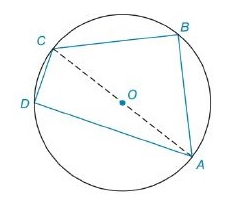Chapter 8.2, Problem 27EElementary Geometry For College St...

7th Edition
Alexander + 2 others
ISBN: 9781337614085

Solutions

Chapter
SectionElementary Geometry For College St...

7th Edition
Alexander + 2 others
ISBN: 9781337614085
Textbook Problem

In Exercises 27 to 30, find the area of the figure shown. Given: In ⊙ O , O A = 5 , B C = 6 , a n d C D = 4 Find: A A B C DTo determine

To Find:

The area of the quadrilateral ABCD inscribed by the circle with centre 'O'.

Explanation

Formula Used:

Angle subtended by a diameter on any point of circle is 90°.

Pythagorean theorem for the right angle triangle ABC for the hypotenuse AC,

AC2=AB2+BC2.

Area of right triangle =12×b×h

Calculation:

Consider the quadrilateral ABCD.

This quadrilateral can be divided into two triangles. That is, ABC and ADC.

By the angle subtended in a diameter of the circle theorem, it makes 90° at the mD and mB.

It is given that OA=5=radius, BC=6 and CD=4.

As radius is 5, the diameter is twice the radius.

CA=10

Consider the ABC,

Here BC=6 and CA=10. Applying Pythagorean theorem,

CA2=AB2+BC2

102=AB2+62

AB2=100-36

AB=8 units

Still sussing out bartleby?

Check out a sample textbook solution.

See a sample solution

The Solution to Your Study Problems

Bartleby provides explanations to thousands of textbook problems written by our experts, many with advanced degrees!

Get Started

Polynomial Inequalities Solve the inequality. 94. x3 3x2 4x + 12 0

Precalculus: Mathematics for Calculus (Standalone Book)

In Exercises 1-6, solve for y. x3y+2y=1

Calculus: An Applied Approach (MindTap Course List)

Solve the equations in Exercises 126. 10x(x2+1)4(x3+1)510x2(x2+1)5(x3+1)4=0

Finite Mathematics and Applied Calculus (MindTap Course List)

0 1 2 ∞

Study Guide for Stewart's Single Variable Calculus: Early Transcendentals, 8th

True or False: is monotonic.

Study Guide for Stewart's Multivariable Calculus, 8th